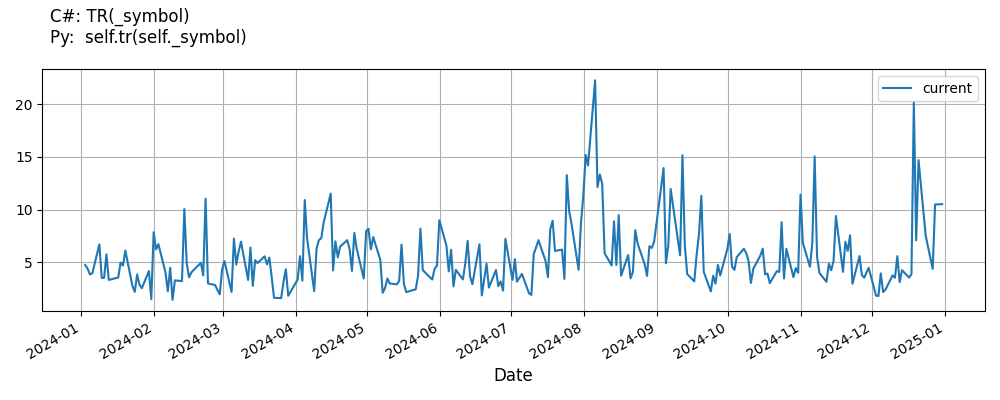# Supported Indicators

## True Range

### Introduction

This indicator computes the True Range (TR). The True Range is the greatest of the following values: value1 = distance from today's high to today's low. value2 = distance from yesterday's close to today's high. value3 = distance from yesterday's close to today's low.

To view the implementation of this indicator, see the LEAN GitHub repository.

### Using TR Indicator

To create an automatic indicators for TrueRange, call the TR helper method from the QCAlgorithm class. The TR method creates a TrueRange object, hooks it up for automatic updates, and returns it so you can used it in your algorithm. In most cases, you should call the helper method in the Initialize method.

public class TrueRangeAlgorithm : QCAlgorithm
{
private Symbol _symbol;
private TrueRange _tr;

public override void Initialize()
{
_tr = TR(_symbol);
}

public override void OnData(Slice data)
{
{
// The current value of _tr is represented by itself (_tr)
// or _tr.Current.Value
Plot("TrueRange", "tr", _tr);

}
}
}
class TrueRangeAlgorithm(QCAlgorithm):
def Initialize(self) -> None:
self.tr = self.TR(self.symbol)

def OnData(self, slice: Slice) -> None:
# The current value of self.tr is represented by self.tr.Current.Value
self.Plot("TrueRange", "tr", self.tr.Current.Value)



The following reference table describes the TR method:

### TR()1/1

            TrueRange QuantConnect.Algorithm.QCAlgorithm.TR (
Symbol                                symbol,
*Nullable<Resolution>           resolution,
*Func<IBaseData, IBaseDataBar>  selector
)


Creates a new TrueRange indicator.

If you don't provide a resolution, it defaults to the security resolution. If you provide a resolution, it must be greater than or equal to the resolution of the security. For instance, if you subscribe to hourly data for a security, you should update its indicator with data that spans 1 hour or longer.

You can manually create a TrueRange indicator, so it doesn't automatically update. Manual indicators let you update their values with any data you choose.

Updating your indicator manually enables you to control when the indicator is updated and what data you use to update it. To manually update the indicator, call the Update method with a TradeBar, or QuoteBar. The indicator will only be ready after you prime it with enough data.

public class TrueRangeAlgorithm : QCAlgorithm
{
private Symbol _symbol;
private TrueRange _tr;

public override void Initialize()
{
_tr = new TrueRange();
}

public override void OnData(Slice data)
{
if (data.Bars.TryGeValue(_symbol, out var bar))
{
_tr.Update(bar);
}

{
// The current value of _tr is represented by itself (_tr)
// or _tr.Current.Value
Plot("TrueRange", "tr", _tr);

}
}
}
class TrueRangeAlgorithm(QCAlgorithm):
def Initialize(self) -> None:
self.tr = TrueRange()

def OnData(self, slice: Slice) -> None:
bar = slice.Bars.get(self.symbol)
if bar:
self.tr.Update(bar)

# The current value of self.tr is represented by self.tr.Current.Value
self.Plot("TrueRange", "tr", self.tr.Current.Value)



To register a manual indicator for automatic updates with the security data, call the RegisterIndicator method.

public class TrueRangeAlgorithm : QCAlgorithm
{
private Symbol _symbol;
private TrueRange _tr;

public override void Initialize()
{
_tr = new TrueRange();
RegisterIndicator(_symbol, _tr, Resolution.Daily);
}

public override void OnData(Slice data)
{
{
// The current value of _tr is represented by itself (_tr)
// or _tr.Current.Value
Plot("TrueRange", "tr", _tr);

}
}
}
class TrueRangeAlgorithm(QCAlgorithm):
def Initialize(self) -> None:
self.tr = TrueRange()
self.RegisterIndicator(self.symbol, self.tr, Resolution.Daily)

def OnData(self, slice: Slice) -> None:
# The current value of self.tr is represented by self.tr.Current.Value
self.Plot("TrueRange", "tr", self.tr.Current.Value)



The following reference table describes the TrueRange constructor:

### TrueRange()1/2

            TrueRange QuantConnect.Indicators.TrueRange (

)


Initializes a new instance of the TrueRange class using the specified name.

### TrueRange()2/2

            TrueRange QuantConnect.Indicators.TrueRange (
string  name
)


Initializes a new instance of the TrueRange class using the specified name.

### Visualization

The following image shows plot values of selected properties of TrueRange using the plotly library.You can also see our Videos. You can also get in touch with us via Discord.